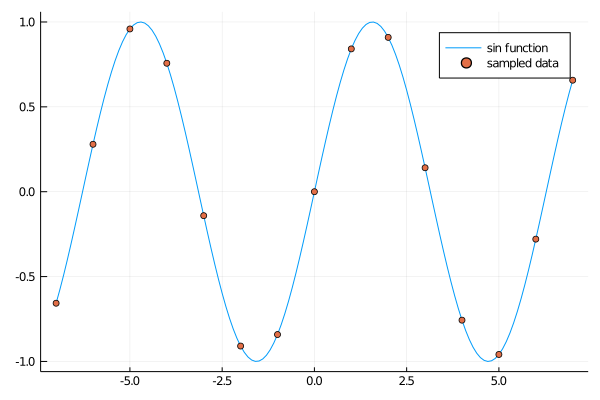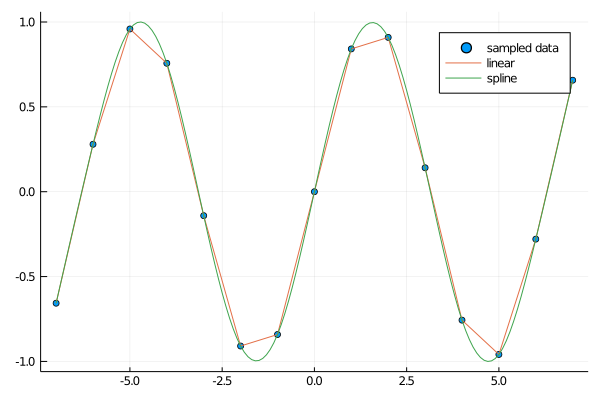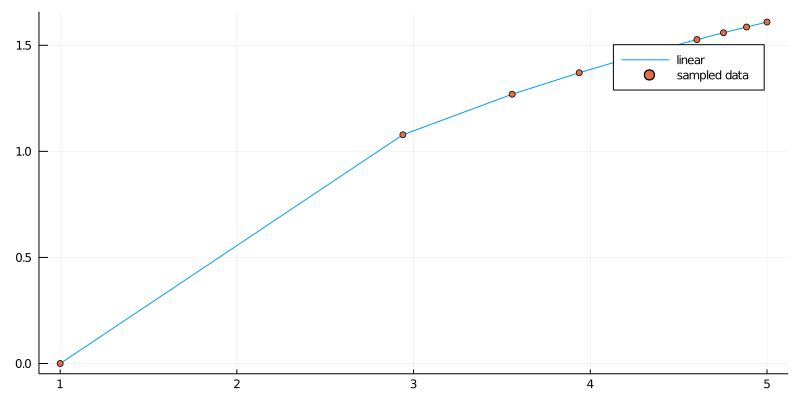# General Purpose Packages¶

## Overview¶

Julia has both a large number of useful, well written libraries and many incomplete poorly maintained proofs of concept.

A major advantage of Julia libraries is that, because Julia itself is sufficiently fast, there is less need to mix in low level languages like C and Fortran.

As a result, most Julia libraries are written exclusively in Julia.

Not only does this make the libraries more portable, it makes them much easier to dive into, read, learn from and modify.

In this lecture we introduce a few of the Julia libraries that we’ve found particularly useful for quantitative work in economics.

Also see data and statistical packages and optimization, solver, and related packages for more domain specific packages.

### Setup¶

In :
using InstantiateFromURL
# optionally add arguments to force installation: instantiate = true, precompile = true
github_project("QuantEcon/quantecon-notebooks-julia", version = "0.8.0")

In :
using LinearAlgebra, Statistics
using Interpolations, Plots, LaTeXStrings, ProgressMeter


## Numerical Integration¶

Many applications require directly calculating a numerical derivative and calculating expectations.

A high accuracy solution for calculating numerical integrals is QuadGK.

In :
using QuadGK
@show value, tol = quadgk(cos, -2π, 2π);

(value, tol) = quadgk(cos, -2π, 2π) = (-1.5474478810961125e-14, 5.7846097329025695e-24)


This is an adaptive Gauss-Kronrod integration technique that’s relatively accurate for smooth functions.

However, its adaptive implementation makes it slow and not well suited to inner loops.

In :
using FastGaussQuadrature
x, w = gausslegendre( 100_000 ); # i.e. find 100,000 nodes

# integrates f(x) = x^2 from -1 to 1
f(x) = x^2
@show w ⋅ f.(x); # calculate integral

w ⋅ f.(x) = 0.6666666666666667


The only problem with the FastGaussQuadrature package is that you will need to deal with affine transformations to the non-default domains yourself.

Alternatively, QuantEcon.jl has routines for Gaussian quadrature that translate the domains.

In :
using QuantEcon

x, w = qnwlege(65, -2π, 2π);
@show w ⋅ cos.(x); # i.e. on [-2π, 2π] domain

w ⋅ cos.(x) = -3.0064051806277455e-15


### Expectations¶

If the calculations of the numerical integral is simply for calculating mathematical expectations of a particular distribution, then Expectations.jl provides a convenient interface.

Under the hood, it is finding the appropriate Gaussian quadrature scheme for the distribution using FastGaussQuadrature.

In :
using Distributions, Expectations
dist = Normal()
E = expectation(dist)
f(x) = x
@show E(f) #i.e. identity

# Or using as a linear operator
f(x) = x^2
x = nodes(E)
w = weights(E)
E * f.(x) == f.(x) ⋅ w

E(f) = -6.991310601309959e-18

Out:
true

## Interpolation¶

In economics we often wish to interpolate discrete data (i.e., build continuous functions that join discrete sequences of points).

The package we usually turn to for this purpose is Interpolations.jl.

There are a variety of options, but we will only demonstrate the convenient notations.

### Univariate with a Regular Grid¶

We begin by creating some data points, using a sine function

In :
using Interpolations
using Plots
gr(fmt=:png);

x = -7:7 # x points, coase grid
y = sin.(x) # corresponding y points

xf = -7:0.1:7        # fine grid
plot(xf, sin.(xf), label = "sin function")
scatter!(x, y, label = "sampled data", markersize = 4)

Out:To implement linear and cubic spline interpolation

In :
li = LinearInterpolation(x, y)
li_spline = CubicSplineInterpolation(x, y)

@show li(0.3) # evaluate at a single point

scatter(x, y, label = "sampled data", markersize = 4)
plot!(xf, li.(xf), label = "linear")
plot!(xf, li_spline.(xf), label = "spline")

li(0.3) = 0.25244129544236954

Out:### Univariate with Irregular Grid¶

In the above, the LinearInterpolation function uses a specialized function for regular grids since x is a Range type.

For an arbitrary, irregular grid

In :
x = log.(range(1, exp(4), length = 10)) .+ 1  # uneven grid
y = log.(x) # corresponding y points

interp = LinearInterpolation(x, y)

xf = log.(range(1,  exp(4), length = 100)) .+ 1 # finer grid

plot(xf, interp.(xf), label = "linear")
scatter!(x, y, label = "sampled data", markersize = 4, size = (800, 400))

Out:At this point, Interpolations.jl does not have support for cubic splines with irregular grids, but there are plenty of other packages that do (e.g. Dierckx.jl and GridInterpolations.jl).

### Multivariate Interpolation¶

Interpolating a regular multivariate function uses the same function

In :
f(x,y) = log(x+y)
xs = 1:0.2:5
ys = 2:0.1:5
A = [f(x,y) for x in xs, y in ys]

# linear interpolation
interp_linear = LinearInterpolation((xs, ys), A)
@show interp_linear(3, 2) # exactly log(3 + 2)
@show interp_linear(3.1, 2.1) # approximately log(3.1 + 2.1)

# cubic spline interpolation
interp_cubic = CubicSplineInterpolation((xs, ys), A)
@show interp_cubic(3, 2) # exactly log(3 + 2)
@show interp_cubic(3.1, 2.1) # approximately log(3.1 + 2.1);

interp_linear(3, 2) = 1.6094379124341003
interp_linear(3.1, 2.1) = 1.6484736801441782
interp_cubic(3, 2) = 1.6094379124341
interp_cubic(3.1, 2.1) = 1.6486586594237707


See Interpolations.jl documentation for more details on options and settings.

## Linear Algebra¶

### Standard Library¶

The standard library contains many useful routines for linear algebra, in addition to standard functions such as det(), inv(), factorize(), etc.

Routines are available for

• Cholesky factorization
• LU decomposition
• Singular value decomposition,
• Schur factorization, etc.

See here for further details.

## General Tools¶

### LaTeXStrings.jl¶

When you need to properly escape latex code (e.g. for equation labels), use LaTeXStrings.jl.

In :
using LaTeXStrings
L"an equation: $1 + \alpha^2$"

Out:
an equation: $1 + \alpha^2$

### ProgressMeter.jl¶

For long-running operations, you can use the ProgressMeter.jl package.

To use the package, you simply put a macro in front of for loops, etc.

From the documentation

In :
using ProgressMeter

@showprogress 1 "Computing..." for i in 1:50
sleep(0.1) # some computation....
end

Computing...100%|███████████████████████████████████████| Time: 0:00:05